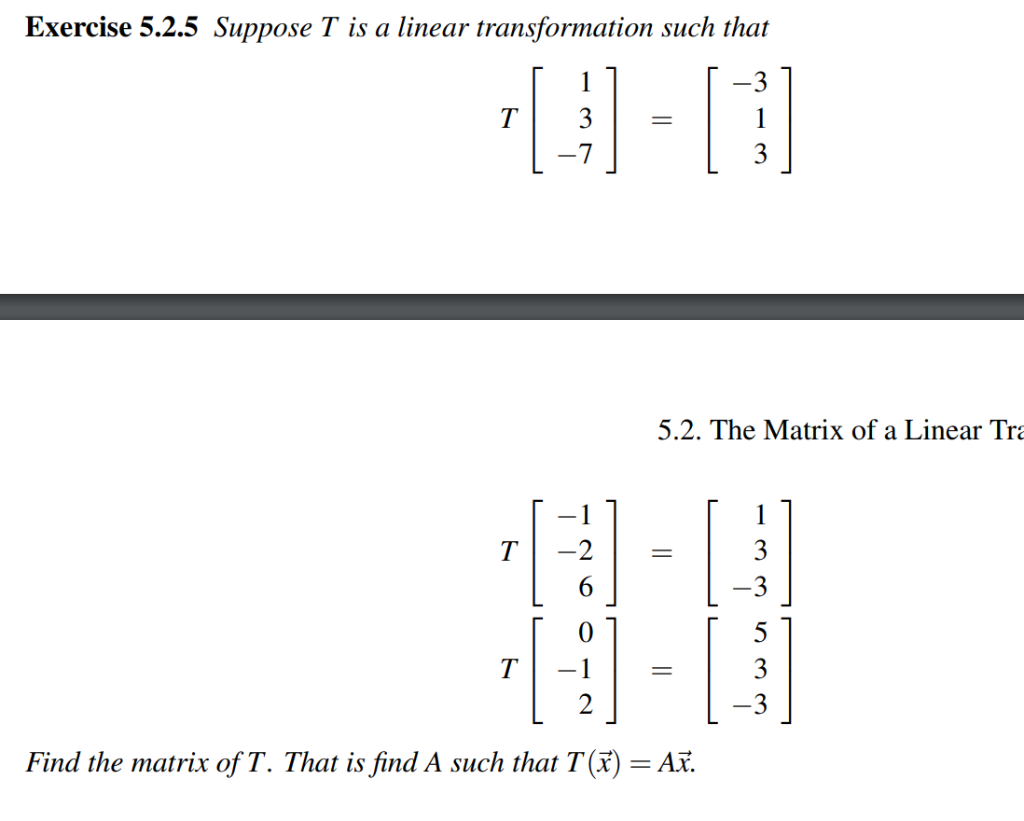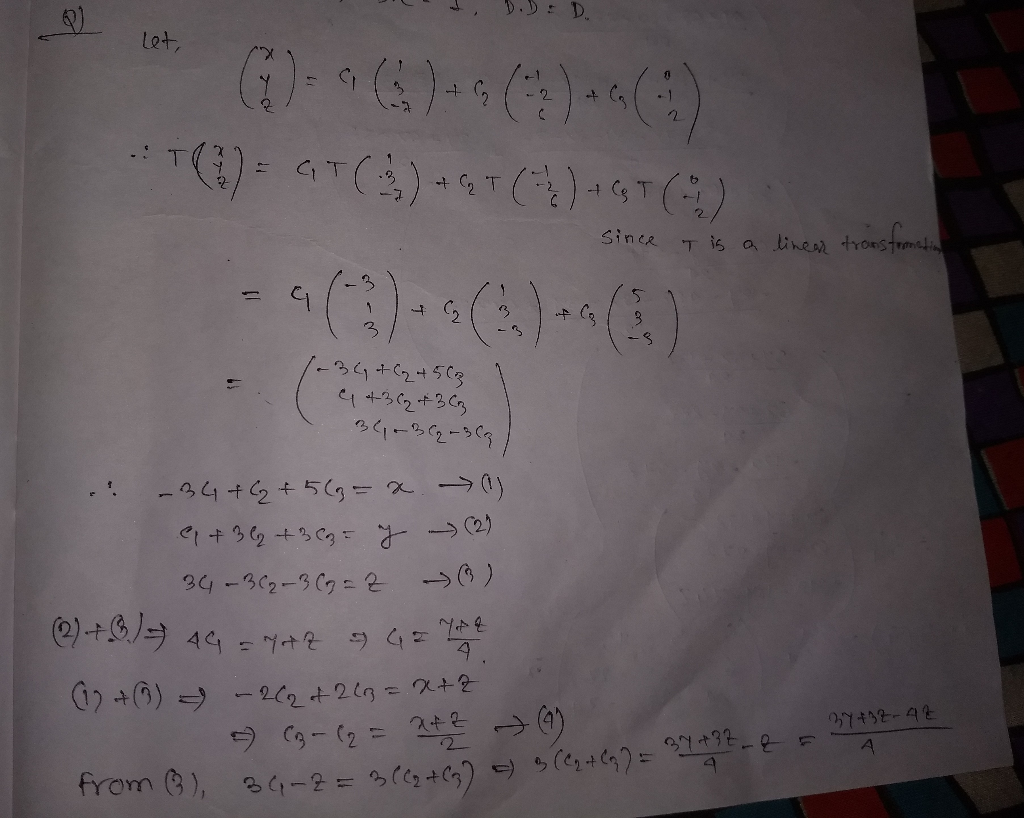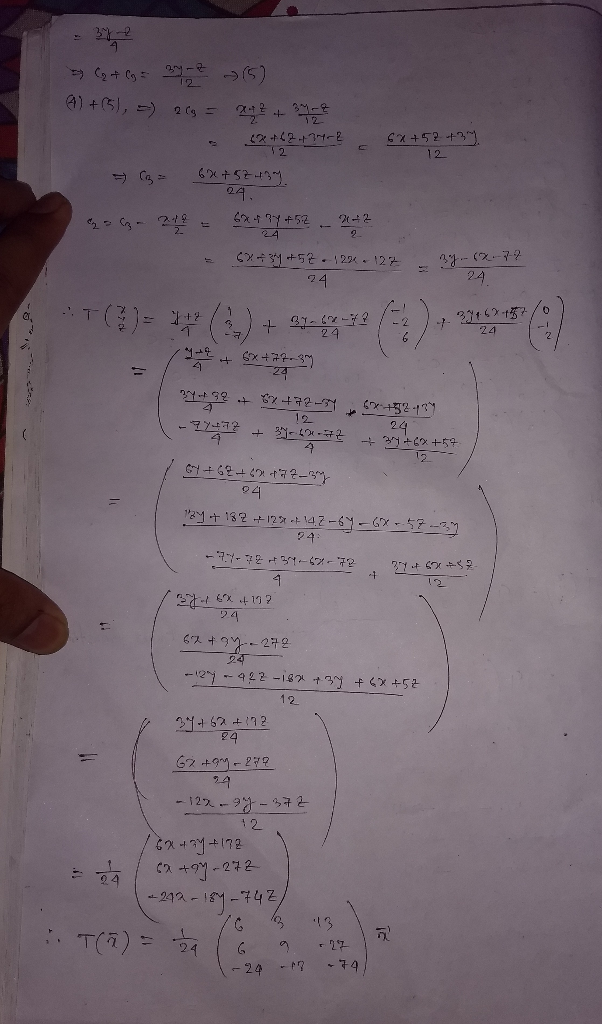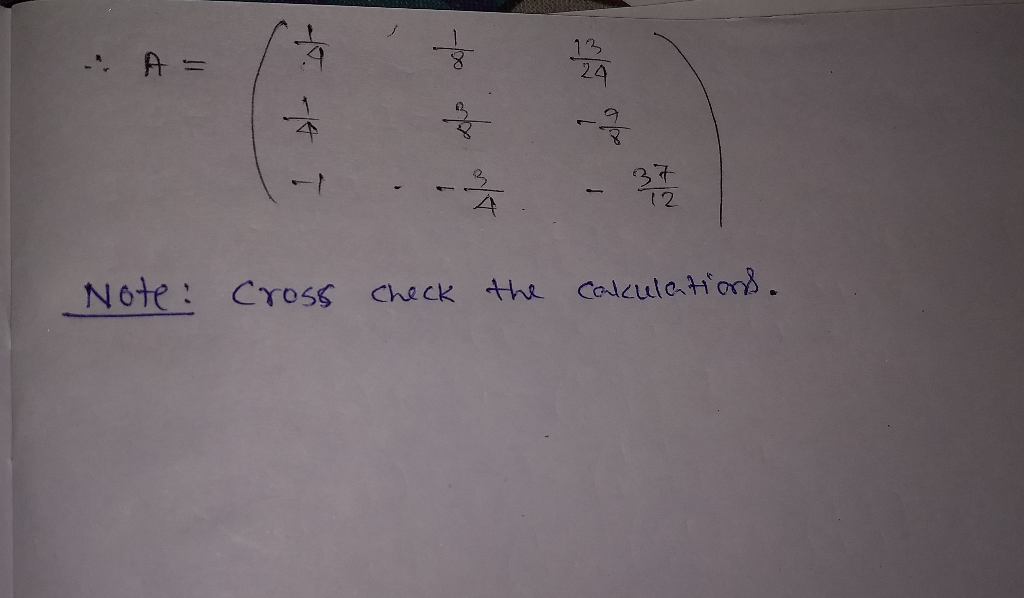Homework Help Question & Answers

# Exercise 5.2.5 Suppose T is a linear transformation such that 7 5.2. The Matrix of a Linear Tr Fi...Exercise 5.2.5 Suppose T is a linear transformation such that 7 5.2. The Matrix of a Linear Tr Find the matrix of T. That is find A such that T()-Aï.##### Add Answer of: Exercise 5.2.5 Suppose T is a linear transformation such that 7 5.2. The Matrix of a Linear Tr Fi...
More Homework Help Questions Additional questions in this topic.

• #### Exercise 34.1 Suppose the filter A: equation '(R) '(R) is governed 2 (a) Compute its impulse response. (b) Compute and represent graphically the step response. Exercise 34.1 Suppose t...

Need Online Homework Help?

Get FREE EXPERT Answers
WITHIN MINUTES
Related Questions